# ISEE Upper Level Quantitative : Pyramids

## Example Questions

### Example Question #1 : Solid Geometry

Pyramid 1 has a square base with sidelength; its height is.

Pyramid 2 has a square base with sidelength; its height is.

Which is the greater quantity?

(a) The volume of Pyramid 1

(b) The volume of Pyramid 2

(b) is greater.

It is impossible to tell from the information given.

(a) is greater.

(a) and (b) are equal.

(b) is greater.

Explanation:

Use the formula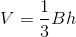on each pyramid.

(a)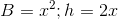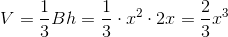(b)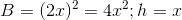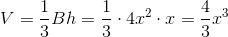Regardless of, (b) is the greater quantity.

### Example Question #2 : Solid Geometry

Which is the greater quantity?

(a) The volume of a pyramid with height 4, the base of which has sidelength 1

(b) The volume of a pyramid with height 1, the base of which has sidelength 2

(a) and (b) are equal.

(a) is greater.

It is impossible to tell from the information given.

(b) is greater.

(a) and (b) are equal.

Explanation:

The volume of a pyramid with heightand a square base with sidelengthis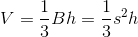.

(a) Substitute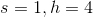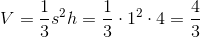(b) Substitute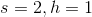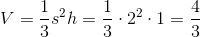The two pyramids have equal volume.

### Example Question #3 : Solid Geometry

Which is the greater quantity?

(a) The volume of a pyramid whose base is a square with sidelength 8 inches

(b) The volume of a pyramid whose base is an equilateral triangle with sidelength one foot

It is impossible to tell from the information given.

(a) and (b) are equal.

(a) is greater.

(b) is greater.

It is impossible to tell from the information given.

Explanation:

The volume of a pyramid is one-third of the product of the height and the area of the base. The areas of the bases can be calculated, but no information is given about the heights of the pyramids. There is not enough information to determine which one has the greater volume.

### Example Question #4 : Solid Geometry

A pyramid with a square base has height equal to the perimeter of its base. Its volume is. In terms of, what is the length of each side of its base?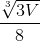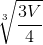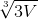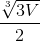Explanation:

The volume of a pyramid is given by the formula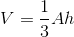whereis the area of its base andis its height.

Letbe the length of one side of the square base. Then the height is equal to the perimeter of that square, so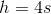and the area of the base is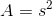So the volume formula becomes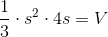Solve for: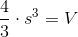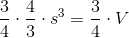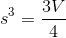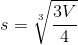### Example Question #301 : Geometry

A pyramid with a square base has height equal to the perimeter of its base. Which is the greater quantity?

(A) Twice the area of its base

(B) The area of one of its triangular faces

(B) is greater

It is impossible to determine which is greater from the information given

(A) and (B) are equal

(A) is greater

(B) is greater

Explanation:

Since the answer is not dependent on the actual dimensions, for the sake of simplicity, we assume that the base has sidelength 2. Then the area of the base is the square of this, or 4.

The height of the pyramid is equal to the perimeter of the base, or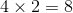. A right triangle can be formed with the lengths of its legs equal to the height of the pyramid, or 8, and one half the length of a side, or 1; the length of its hypotenuse, which is the slant height, is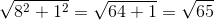This is the height of one triangular face; its base is a side of the square, so the length of the base is 2. The area of a face is half the product of these dimensions, or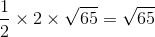Since twice the area of the base is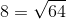, the problem comes down to comparing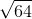and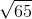; the latter, which is (B), is greater.

### Example Question #311 : Geometry

A pyramid with a square base and a cone have the same height and the same volume. Which is the greater quantity?

(A) The perimeter of the base of the pyramid

(B) The circumference of the base of the cone

(A) and (B) are equal

(A) is greater

(B) is greater

It is impossible to determine which is greater from the information given

(A) is greater

Explanation:

The volume of a pyramid or a cone with heightand base of areaisso in both cases, the area of the base is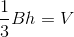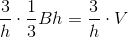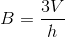Since the pyramid and the cone have the same volume and height, their bases has the same area.

The length of one side of the square base of the pyramid is the square root of this, or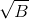, and the perimeter is four times this, or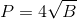.

The radius and the area of the base of the cone are related as follows: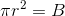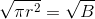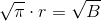Multiply both sides by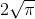to get: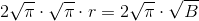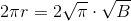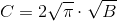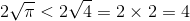, so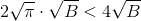, and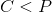The perimeter of the base of the pyramid, which is (A), is greater than the circumference of the base of the cone.